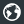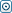Forex Stock Exchange Forum
Would you like to react to this message? Create an account in a few clicks or log in to continue.

# WSS Forex Instrument - VertexFXOmSaiTech
Number of messages : 62
Points : 2186
Date of Entry : 2016-05-08
Year : 42
Residence Country : India##WSS Forex Instrument - VertexFX

Sat Oct 15, 2016 6:11 am
The W S S Forex Instrument Expert Advisor is a pivot-based VertexFX client-side script that places trades based on Camarilla (Daily) pivot levels.

Camarilla Pivots based on Daily charts are very powerful in identifying Support and Resistance levels. When the price crosses these levels, the likelihood of a strong breakout is very high. This Expert Advisor uses this concept to identify profitable trading opportunities.

The EA calculate the Camarilla Pivot by adding the previous Day’s Open, High, Low and Close, and then dividing this sum by 4.

The EA then proceed to calculate the four levels, namely L W B, L B B, L W R and L R R as follows:

L W B is calculated by adding the value of METRIC to the Camarilla Pivot calculated in the first step. The value of L B B is calculated by adding twice the value of METRIC to the Camarilla Pivot calculated in the first step.

The value of L W R is calculated by subtracting the value of METRIC from the Camarilla Pivot calculated in the first step. Finally, the value of L R R is calculated by subtracting twice the value of METRIC from the Camarilla Pivot calculated in the first step.

These four levels are the trading bands which must be first broken in order for a breakout to happen. These bands occur within the range of the previous Day.

Finally, the EA calculates buy and sell levels as follows,

The BUY_LEVEL is the maximum value of L R R and 0.55 times the previous Day’s Low subtracted from the sum of previous Day’s Close and High.

The BUY_LEVEL is the minimum value of L R R and 0.55 times the difference between previous Day’sHigh and Low subtracted from the previous Day’s Close.

The Expert Advisor then places a BUY STOP order at the BUY_LEVEL and a SELL_STOP order at the SELL_LEVEL. If either order is executed, then the pending opposite order is deleted.

The Expert Advisor trades only between the START_HOUR_FROM and END_HOUR_FROM. Pending orders are placed when the session is active. Once the session becomes inactive, pending orders and open positions are closed.
The Expert Advisor also employs a trailing stop with a distance specified by TRAIL for track and protect profitable positions.

Permissions in this forum:
You cannot reply to topics in this forum